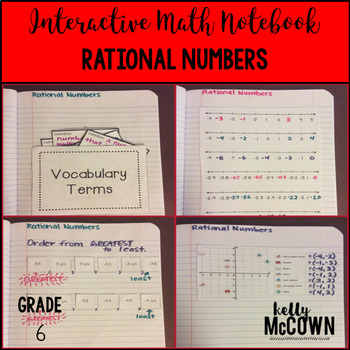## Friday, September 16, 2016

### Teaching Rational Numbers {Grades 6 & 7}

Oh how I love a rational number! In mathematics, a rational number is any number that can be expressed as the quotient or fraction x/y of two integers, a numerator x and a non-zero denominator y. Every integer is a rational number! That's a lot of numbers. To get students engaged in Rational Numbers I have used many different activities. Here are some of my favorite activities for teaching Rational Numbers.1. Math Stations for Rational Numbers

This math station activity is intended to help students understand a rational number as a point on the number line. Students will also extend number line diagrams and coordinate axes. Students will also understand ordering and absolute value of rational numbers.
Included are:
-6 different stations to engage students
-Teacher facilitated activity for 60-90 minutes of classroom time
-Stations include: 1. Vocabulary 2. Technology (Need classroom computers & internet access to ixl.com) 3. Practice of Rational Numbers 4. Additional Practice of Rational Numbers 5. Real World applications of Rational Numbers 6. Word problems of Rational Numbers
-Student Station Guide helps students record their answers2. Interactive Math Notebook Activities for Rational Numbers

These interactive notebook activities are intended to help students understand a rational number as a point on the number line. Students will also extend number line diagrams and coordinate axes. Students will also understand ordering and absolute value of rational numbers.
Included are:
-4 different Rational Numbers activities to engage students
-Teacher facilitated activity for 60-90 minutes of classroom time
-Activities include: Vocabulary, Practice of Rational Numbers, Ordering Rational Numbers, and Creating Number Lines with Rational Numbers
-Examples of Completed Interactive Math Notebook Activities are included

I hope these activities help you to jump start into Rational Numbers. Remember to actively engage your students in the learning process and they will own their knowledge!